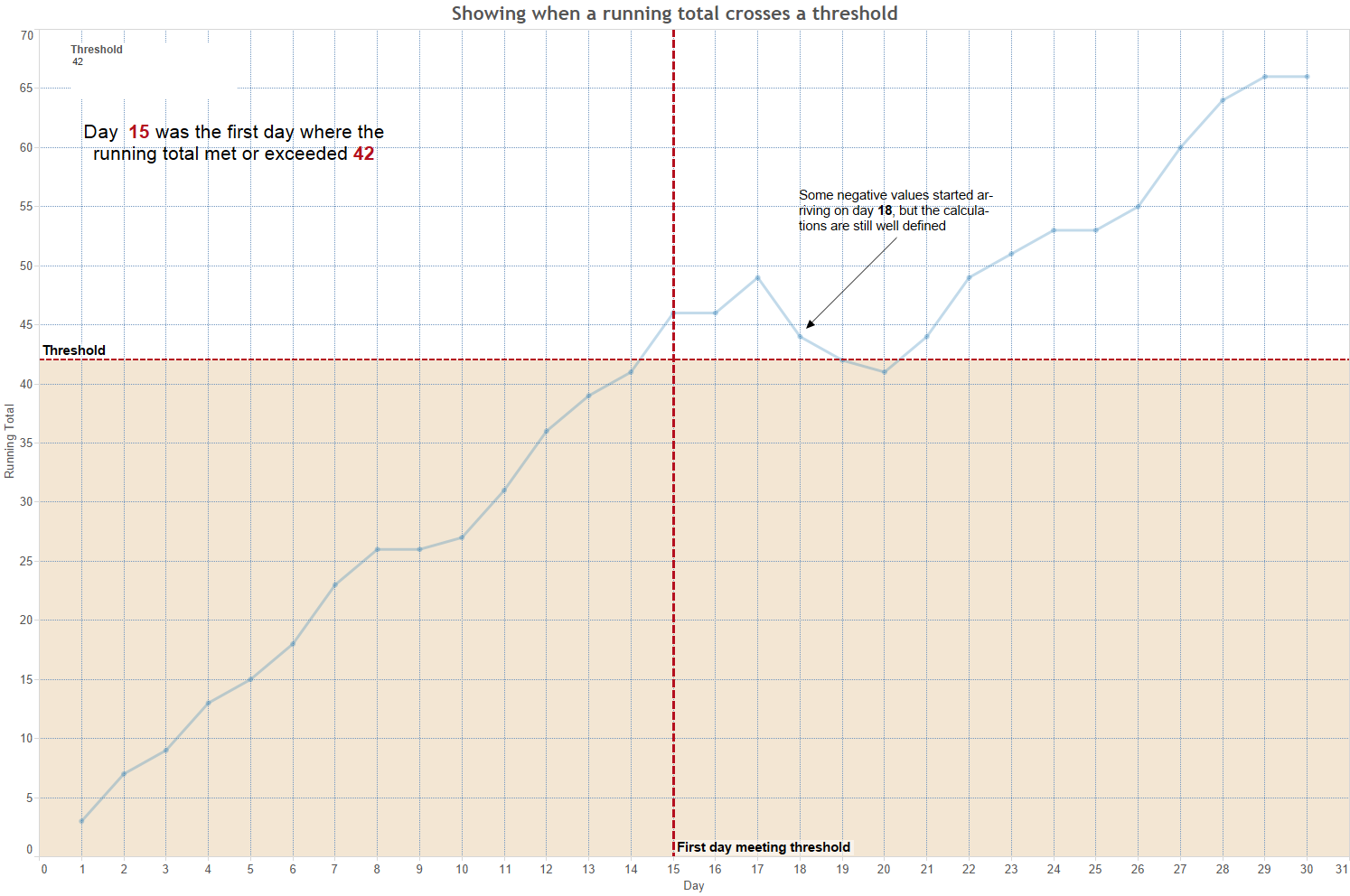# Return Value first time condition is met

1 view
in BI

I have a calculation, and I only want to return the leftmost value ie, the first time the condition is met.In the images, it shows 95% 96%, etc I only care for column 5 where the condition of 95 is met.

Ok, so the percentages are calculated this way.

ROUND( RUNNING_SUM( SUM( [days_to_close_cnt] ) )/ SUM([newo_dt_cnt]),2 )

Using and the "Days to Close" gives me the column information.

The Line graph looks like this.if [% Completed] >= .98  and  [% Completed] < 1 then INT( 98 )

elseif [% Completed] = 1  then INT(  1 )

elseif  [% Completed] >= .95  and [% Completed] < .98 then INT( 95 )

elseif  [% Completed] < .95 and [% Completed] >= .0 then INT(  0 )

end

Is that calc I am using to color in this case.by (46.1k points)
• I think you are trying to tell when a running total crosses a threshold. Doing that in Tableau requires table calculations which operate on the aggregated values that have been returned from the data source.

• In order to illustrate how to approach the issue,I will put together an example viz.

• Through the link above, try hovering over some of the data points to see the tooltips for detail, and incrementally increasing the threshold to see how the calculation behaves in the live version.

• In order to see some of the intermediate results, you can also click on the table tab. In case you can't access the dynamic one linked above, Here's a static snapshot• In a nutshell, you can define a boolean calculated field to tell whether the running total meets the threshold on a particular day and then use window_min() to calculate the first day that meets the threshold. For more details, download the workbook and check out the calculated fields to understand how it works.

• Table calcs are powerful but are also the feature in Tableau that takes the most time to get your head around. So break things down and tackle them in small bites instead of trying to write complex table calcs all at once. Luckily, there are shortcuts (quick table calcs) for the most common cases. Be aware that specifying the partitioning and addressing fields (i.e., compute using) for a table calc is as important as writing the formula.

• Finally, as an aside, your calculated field can be written more efficiently as:

if [% Completed] < .95 then 0

elseif [% Completed] < .98 then 95

elseif [% Completed] < 1 then 98

else 1

end